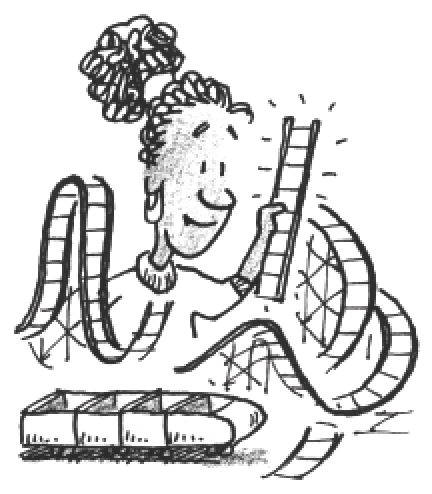### Home > APCALC > Chapter 2 > Lesson 2.1.2 > Problem2-23

2-23.

Kristin is designing a model that will represent the path of a roller coaster. She has determined the beginning and the end parts of the track, but needs to write a formula for the middle section that will join the other segments. She decides that she wants one peak in this middle section, not including its boundaries. What values of $a$ and $b$ will make her model, given below, a continuous function? To help you visualize this, use the
$f ( x ) = \left\{ \begin{array} { l l } { - 2 \operatorname { cos } x + 3 } & { \text { for } x \leq 0 } \\ { a \operatorname { cos } ( b x ) - 2 } & { \text { for } 0 < x \leq 2 \pi } \\ { - \operatorname { cos } ( 2 x ) - 4 } & { \text { for } x > 2 \pi } \end{array} \right.$

Sara's roller coaster needs to be continuous. That means that the $y$-values at the boundary points $(x = 0 \text { and } x = 2π)$ need to agree from the left and the right.

Determine the $y$-value of the roller coaster at $x = 0: −2\cos(0) + 3 = 1$

Next, determine the $y$-value of the roller coaster at $x = 2\pi: −{\cos}(2(2\pi)) −4 = −5$

We are ready to find the equation of the middle piece of the piecewise function. This piece has two unknowns, $a$ and $b$. Fortunately, in Step 2 and Step 3, we found two values that exist on the middle equation, if the piecewise is continuous.
Write and solve a system for $a$ and $b$:
$1 = a{\cos}(bx) −2 \text{ when } x = 0$
$−5 = a{\cos}(bx) − 2 \text{ when } x = 2\pi$Use the eTool below to dynamically adjust the graph.
Click the link at right for the full version of the eTool: Calc 2-23 HW eTool